﻿ Fractions Worksheets and Activities (Key Stage 1 and Key Stage 2)
 HOME ABOUT US SHOP PRIVACY CONTACT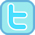# Fractions Worksheets and Resources

### Free worksheets, interactivities and other resources to support teaching and learning about fractions.

 Mad Maths Minutes - Fluency and Timed Drill Halves / Doubles (within 24) Halves / Doubles (multiples of 5) Halves (within 50) Step-by-Step Halves (within 100) Step-by-Step Quarters (within 24) Fraction Walls Quarters (within 100) Fraction Walls Unit Fractions of Quantities Halves and Quarters of Shapes Non-Unit Fractions of Shapes Recognising Tenths
 Halves and Quarters PowerPoint Starters, Plenaries and Problems Is Snappy correct? Adding Halves Half Price Sale Quarter Wall Quarters (Equivalence)
 Halves and Quarters Worksheets & Printables (.pdf) Finding Half of Shapes (Sheet 1) Finding Half of Shapes (Sheet 2) Finding Half of Shapes (Sheet 3) Finding Half of Shapes (Sheet 4) 32 Half and Quarter Challenge Cards Finding Half of a Set (Sheet 1) Finding Half of a Set (Sheet 2) Finding Half or Quarter of a Set (Sheet 1) Finding Half or Quarter of a Set (Sheet 2) Finding Quarter of Shapes (Sheet 1) Finding Quarter of Shapes (Sheet 2) Finding Quarter of Shapes (Sheet 3) Half Consolidation Sheet How Many Halves/Quarters? More than, less than or equal to half More than, less than or equal to half (2) Quarter Consolidation Sheet Shade the Fraction - whole, half, quarters What fraction is shaded?
 Our Tablet-Friendly Halves and Quarters Activities Half or Not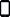Half, More or LessWhole, Half or Quarters#### Interactive Fraction Activities (Fractions of Shapes)Identifying Unit Fractions of Shapes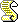Fractions of shapes (multiple parts of whole)#### Interactive Fraction Activities (Fractions of sets / numbers)Find half by sharingHalving to half of 10 Quick RecallHalving to half of 20 Quick RecallFinding Quarter/Three-Quarters by SharingFinding Unit Fractions by SharingFinding Halves, Quarters and Thirds by SharingFind fractions by sharing (multiple parts of whole)Find Quarters by Halving then Halving Again#### Interactive Fraction Activities (Equivalence and Equivalent Fractions)Equivalent to a Half or Not InteractiveEquivalent Fractions Memory SquaresEquivalent Fractions Fill in the Blanks (Unit Fractions)Equivalent Fractions Fill in the Blanks (Unit & Non-Unit Fractions)#### Other Interactive Fraction Activities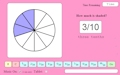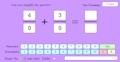Recognising Tenths InteractiveRecognising HundredthsConverting between tenths and hundredthsAdding Fractions (Same Denominator, Within 1) InteractiveSubtracting Fractions (Same Denominator, Within 1) InteractiveAdding/Subtracting Fractions (Same Denominator, Within 1) InteractiveAdding Fractions (change a denominator, within 1)Adding Fractions (Find a Common Denominator)Subtracting Fractions (change a denominator, within 1)Subtracting Fractions (change both denominators, within 1)Compare Fractions (Unit or Same Denominator)Comparing Fractions with Different Denominators (Simple)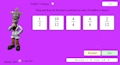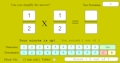Ordering Fractions (Different Denominators)Comparing Improper Fractions and Mixed NumbersSimplifying Fractions (to unit fractions)Simplifying Fractions (up to 24ths)Multiplying Proper Fractions